# Mathematics

Four credits of mathematics are required of all students to fulfill graduation requirements.  Many colleges require completion of Trigonometry.  In these courses, students develop skills in problem solving and critical thinking. Placement within mathematics courses are made by teacher recommendation.  When considering recommendations, successful completion” of a course would typically be indicated by a grade of “C” or better.  Students are encouraged to purchase their own calculators.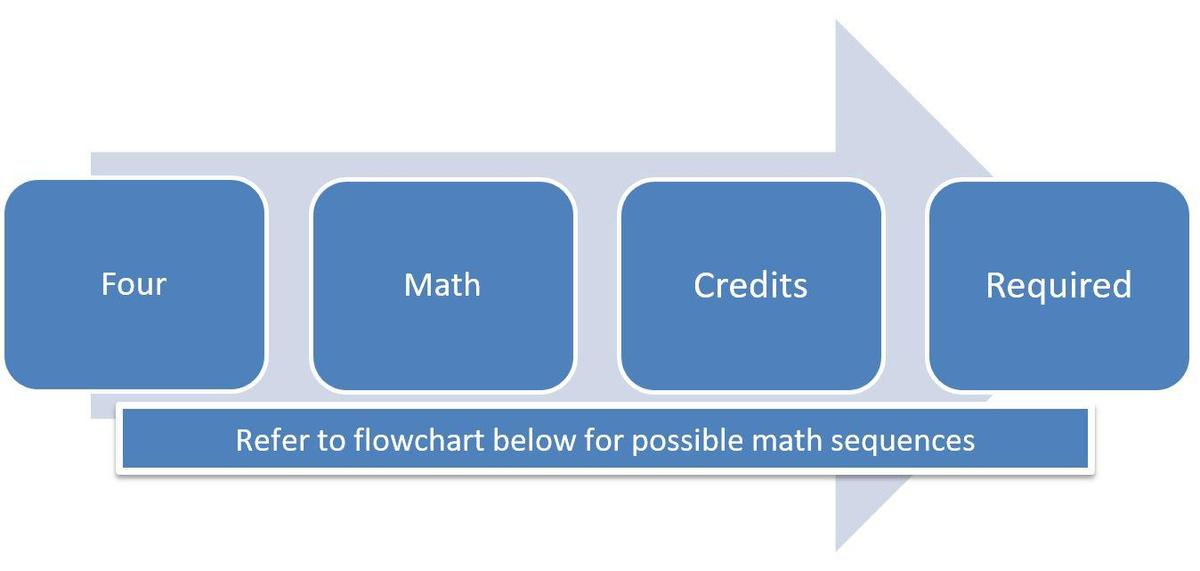The following flow chart illustrates the typical progressions in mathematics.
Placement recommendations are considered each year.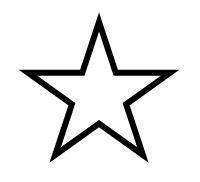= possible starting points for 9th grade students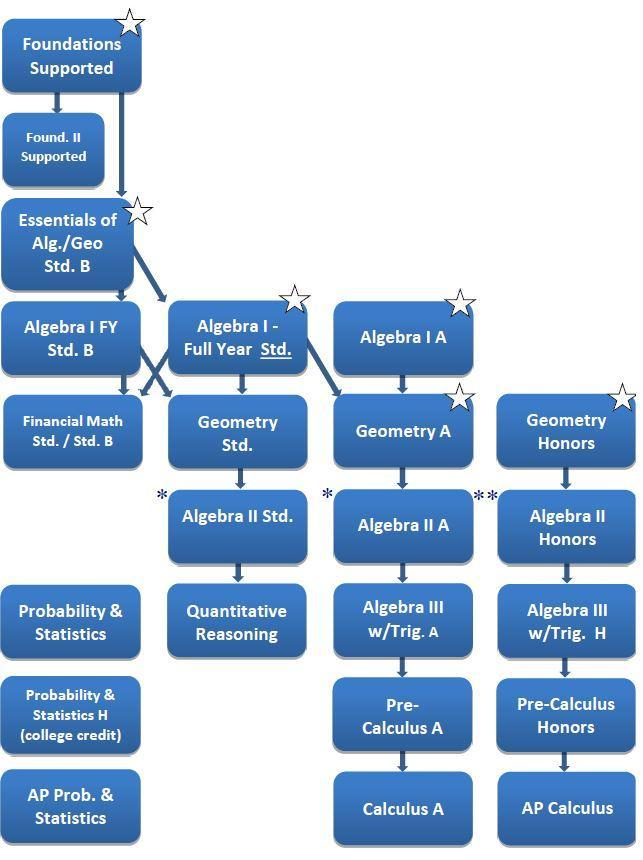Note: Students pursuing the honor-level math track should take two courses Sophomore year.
* Probability and Statistics may be taken any time after completion of Algebra II
**AP Statistics may be taken any time after completion of Algebra II (with grade of B or better)

Foundations of Math I (Supt)             0311
In this course, students increase their basic math skills by reviewing key competencies such as performing basic operations with whole numbers, decimals, and fractions as well as using ratios, proportions, and percents.  Students practice these skills using worksheets, computers, and group work.  They are assessed using class work, homework, quizzes, masteries and projects.  Students take this course  if they need extra preparation before Algebra.
Prerequisite: Teacher recommendation only

Essentials of Algebra & Geometry (Std. B)               0320
In this course, students strengthen their skills required for success in Algebra I as well as begin to study key Algebra I competencies. Students will also learn basic competencies of Geometry. These competencies include performing operations on rational numbers, solving linear equations, applying basic geometric vocabulary,  finding perimeter and area of two-dimensional figures, and solving problems using the Pythagorean Theorem. Students practice these skills using worksheets, activities, and group work. They are assessed using class work, homework, quizzes, masteries and projects.
Prerequisite: Completion of Foundations I and Teacher recommendation only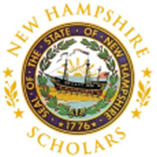Algebra I - Full Year (Std.)                       0326                  (Standard Level NH Scholars eligible)
In this year long course, students learn the same competencies found in the semester Algebra I curriculum as well as key Introduction to Algebra competencies. These competencies include performing order of operations on rational numbers; solving linear and absolute value equations, inequalities, and word problems; writing and graphing linear equations; solving systems of equations; and evaluating functions. Students practice these skills using work-sheets, group work, hands-on activities, and experimental labs. Students are assessed using class assignments, quizzes, weekly masteries, and projects.
Prerequisite: Completion of the 8th grade Intro to Algebra course or the High School Essentials course and Teacher recommendation only

Algebra I - Full Year (Std. B)                    0330
In this year long course, students learn the same competencies found in the semester Algebra I curric-ulum as well as key Introduction to Algebra competencies. These competencies include performing order of operations on rational numbers; solving linear and absolute value equations, inequalities, and word problems; writing and graphing linear equations; solving systems of equations; and evaluating functions. Students practice these skills using work-sheets, group work, hands-on activities, and experimental labs. Students are assessed using class assignments, quizzes, weekly masteries, and projects.
Prerequisite: Completion of Essentials course and Teacher recommendation onlyAlgebra I (Std. A)                     0328
In this course, students learn all of the competencies in a traditional Algebra I curriculum.  These competencies include solving linear and absolute value equations, inequalities and word problems; writing and graphing linear equations; solving systems of equations; and evaluating functions.  Students practice these skills using worksheets, group work, hands-on activities, and experimental labs.  Students are   assessed using assignments, quizzes, masteries, and projects.
Prerequisite: Completion of 8th grade Intro to Algebra  with a B or better or Teacher Recommendation

Financial Math (Std. B)                      0307
Financial Math (Standard)                 0308
In this course, students strengthen those essential math skills needed to make responsible and effective financial decisions. Students will study competencies in financial literacy such as money management, banking services, long and short-term financing, consumer awareness as well as financial planning and investing.  Students practice these skills using projects, activities, and group work. They are assessed using classwork, homework, quizzes, mastery tests and projects.
Prerequisite: Successful completion of Algebra 1Geometry (Std. A)                        0334
Geometry (Honors)                      0335       (Honors & A-level NH Scholars eligible)

Geometry (Std.)                            0333
In this course, students study geometric competencies such as exploring angle relationships both on parallel lines and in triangles; applying the distance, midpoint, and slope formulas; using the properties of triangles; and using the facts of similar figures to solve other problems. Students practice these skills using worksheets, investigations, and group work. They are assessed using class work, homework, quizzes, masteries and projects. This course is offered at four different levels in order to facilitate differentiated instruction.  Honors level students should be accomplished or exemplary complex thinkers.
Prerequisite: Successful completion of Algebra 1Algebra II (Std. A)                      0344
Algebra II (Honors)                   0345      (Honors & A-level NH Scholars eligible)

Algebra II (Std.)                          0338
In this course, students master competencies including simplifying and evaluating advanced algebraic expressions; performing operations with complex numbers and polynomials; solving quadratic equations, and graphing quadratic functions.  Students practice these skills using worksheets, investigations, and group work. Students are assessed using class work, homework, quizzes, masteries and projects.  This course is offered at three different levels in order to facilitate differentiated instruction.  Honors level students should be accomplished or exemplary complex thinkers.
Prerequisite: Successful completion of Algebra 1Algebra III with Trigonometry         (Std. A)                        0365
Algebra III with Trigonometry        (Honors)                       0364
In this course students explore trigonometric  and algebraic competencies including: applying the laws of triangles to real world problems, graphing trigonometric function including polynomials and rationales. Students practice these skills using labs, hands-on activities, worksheets, and group work.  They are assessed using class work, homework, quizzes, masteries and projects.  This course is also offered at the Honors level for the student with the ability and interest  to pursue a faster paced and more in-depth course of study.
Prerequisite: Successful completion of Algebra II and Geometry.Quantitative Reasoning (Std.)               0382
This course is designed to expose students to a wide range of general mathematics.  Problem solving and critical thinking skills, along with the use of technology, will be emphasized and reinforced throughout the course as students become actively involved in solving applied problems.   Topics include: Number Theory and Systems, Functions and Modeling, Finance, Geometry and Measurement, Probability and Statistics and selected topics.
Prerequisite: Completion of Algebra IIPrecalculus (Std. A)                        0374
Precalculus (Honors)                     0369
In this course, students explore a broad range of mathematical topics that expand upon competencies mastered in Algebra II and Trigonometry.  Competencies include graphing conic sections, comparing and contrasting types of functions, solving logarithmic and exponential equations, finding the general term of a sequence, finding the sum of a series, and calculating limits of a function.  Students are assessed using class work, homework, quizzes, masteries and  projects.  This course is also offered at the Honors level for the student with the ability and interest to purse a faster paced and more in-depth course of study.
Prerequisite: Successful completion of Algebra II and TrigonometryCalculus (Std. A)                             0372
In this course, students prepare for the  first semester of college calculus.  Student focus on competencies that include graphing functions both with and without a graphing calculator, reviewing  trigonometric functions, evaluating limits, finding derivatives and integrals of transcendental functions, and exploring basic applications.  Students practice these skills using graphing calculators, investigations, worksheets, and group work.  They are assessed using class work, homework, quizzes, masteries and projects.
Prerequisite:  Successful completion of Precalculus or teacher recommendationProbability & Statistics (Std. A)                   0375
In this course, students learn how to organize, display and analyze data using a variety of statistical techniques and various probability events. The statistical competencies include descriptive statistics, including frequency distributions, standard deviation, and random sampling. The probability competencies include basic probability and binomial distributions. Students are assessed using class work, homework, quizzes, masteries
and projects.
Prerequisite: Successful completion of Algebra II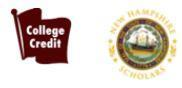AP Calculus [AB] (Honors/AP)                  0370
This college-level course is approved by the College Board as an official Advanced  Placement course, following the curriculum requirements of the AP Calculus AB-level exam.  In this rigorous course, students develop an understanding of calculus and gain experience with its methods and applications.  They explore competencies such as limits and continuity, derivatives, and integrals.  Students practice these skills through both individual and group work.  They are assessed using class work, homework, quizzes, tests and projects.  Students take the Advanced Placement standardized test in May.  With a qualifying score on the AP test, students may earn college credit and/or placement when entering college. As preparation for the rigorous curriculum, students complete a mandatory summer review assignment prior to the first day of class.
Prerequisite: Completion of Precalculus Honors with a grade of B– or higher or teacher recommendation
College Credits: 4 Running Start College Credits are available through Nashua Community College.AP Statistics (Honors/AP)                 0378
This college level course is approved by the College Board as an official AP course, following the curriculum requirements of the AP Statistics exam. The purpose of the AP course in statistics is to introduce students to the major concepts and tools for collecting, analyzing and drawing conclusions from data. Students are exposed to four conceptual themes:  Exploring Data: Describing patterns and departures from patterns; Sampling and Experimentation: Planning and conducting a study; Anticipating Patterns: Exploring random phenomena using probability and simulation; Statistical Inference: Estimating population parameters and testing hypotheses. Students who take the AP Statistics exam may be eligible for college credit and/or advanced placement depending on the college requirements and the exam score. A TI-Nspire calculator is required for this course.
Prerequisite: Completion of Algebra II Honors with a grade of B or higher or teacher recommendationQualifies for NH Scholars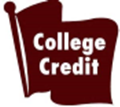Awards College Credit